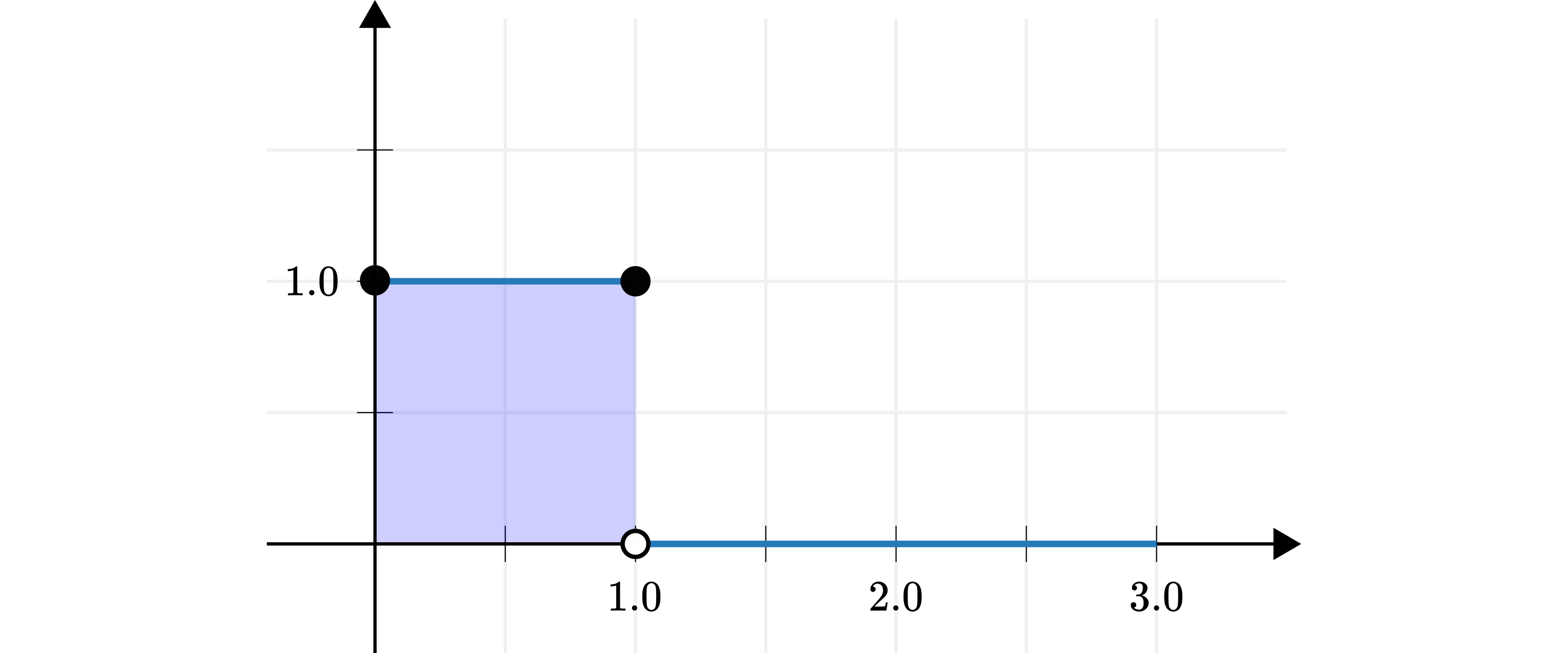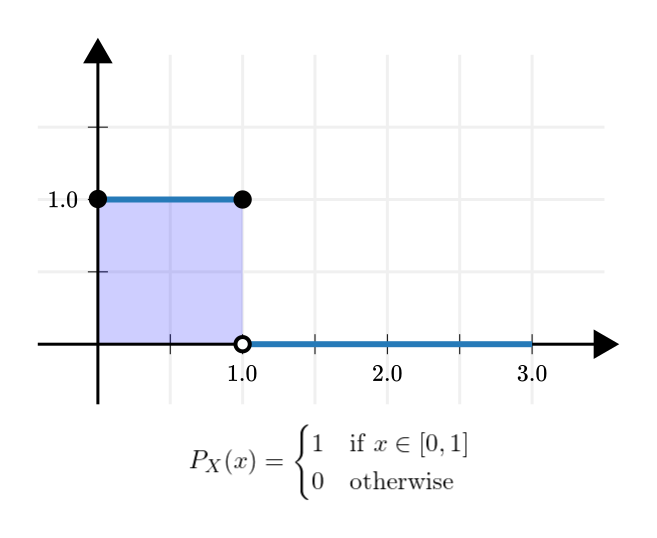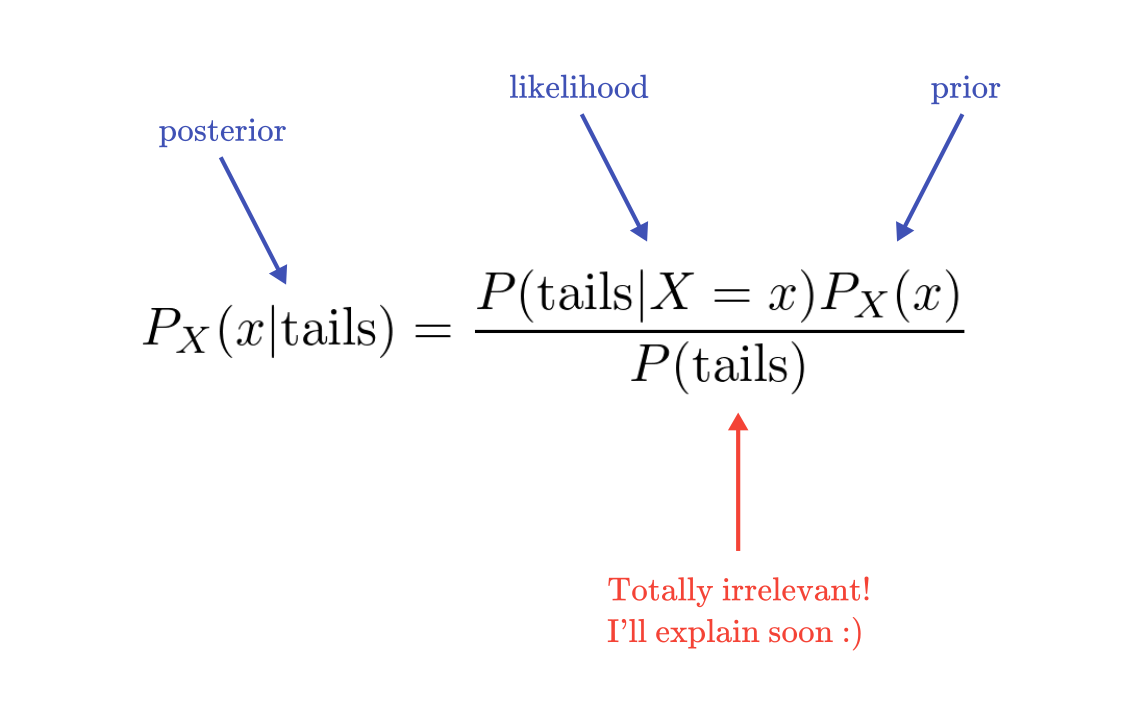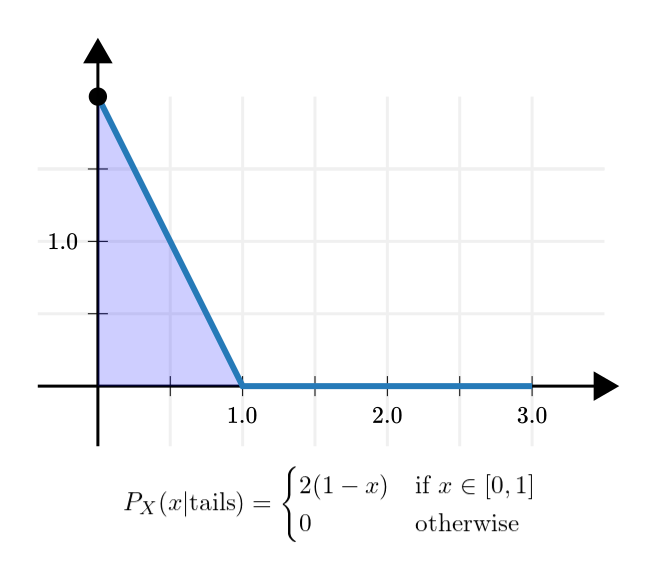# The Bayes formula explained in pure EnglishYou can explain the Bayes formula in pure English. (Even without using any mathematical terminology.)

Despite being overloaded with seemingly complex concepts, it conveys an important lesson about how observations change our beliefs about the world.

$\displaystyle P(B | A) = \frac{P(A | B) P(B)}{P(A)}$

Let's take it apart!

## Updating probabilistic beliefs

Essentially, the Bayes formula describes how to update our models, given new information.

To understand why, we will look at a simple example with a twist: coin tossing with an unfair coin.

Let's suppose that we have a magical coin! It can come up with heads or tails when tossed, but not necessarily with equal probability. The catch is, we don't know the exact probability. So, we have to perform some experiments and statistical estimation to find that out.

To mathematically formulate the problem, we denote the probability of heads with $\textstyle x$, that is,

$\displaystyle P(\text{heads}) = x, \quad x \in [0, 1].$

What do we know about $\textstyle x$? 🤔

At this point, nothing. It can be any number between 0 and 1.

## The Bayesian prior

Instead of looking at $\textstyle x$ as a fixed number, let's think about it as an observation of the experiment $\textstyle X$. To model our (lack of) knowledge about $\textstyle X$, we select the uniform distribution on $[0, 1]$. This is called the prior, as it expresses our knowledge before the experiment.So, suppose that we have tossed our magical coin up, and it landed on tails. How does it influence our model of the coin?

We can tell is that if the probability of heads is some $\textstyle x$, then the likelihood of our experiment resulting in tails is $1-x$:

$\displaystyle P(\text{tails} | X = x) = 1 - x.$

Notice that we want to know the probability distribution with the condition and the event in the other way around: we are curious about our probabilistic model of the parameter, given the result of our previous experiment. This is called the posterior distribution. That is, we are looking for $P_X(x | \text{tails})$.

Now let's put everything together!

## Bayes formula: posterior from the prior

The Bayes formula is precisely what we need, as it expresses the posterior in terms of the prior and the likelihood.It might be surprising, but the true probability of the experiment resulting in tails is irrelevant.

Why? Because it is independent of $\textstyle X$. Also, because we are talking about probability distributions, the integral of the posterior evaluates to 1:

$\int_{0}^{1} P_X(x | \text{tails}) dx = 1.$

Here, the probability of tails is $\textstyle 0.5$, as the law of total probability implies:

$P_X(\text{tails}) = \int_{0}^{1} P(\text{tails} | X = x) P_X(x) dx = \frac{1}{2}.$

(In the general case, integrals like this can be hard to evaluate analytically.)

So, we have our posterior distribution! Notice that it is more concentrated around $x = 0$. (Recall that $\textstyle x$ is the probability of heads.)In other words, this means that if we only saw a single coin toss that resulted in tails, we guess that the coin is biased towards that.

Of course, we can do more and more coin tosses, which can refine the posterior even further. After $\textstyle k$ heads and $n-k$ tails, the posterior will be the so-called Beta distribution.

## The Bayes formula in English

To summarize, here is the Bayes formula in pure English. (Well, sort of.)

posterior ∝ likelihood times prior

Or, in other words, the Bayes formula describes how to update our models, given new observations.

Thus, it plays a fundamental role in probability, statistics, and machine learning. For instance, this is where the famous Mean Squared Error comes from! If you don't believe me, check out my recent post on this!